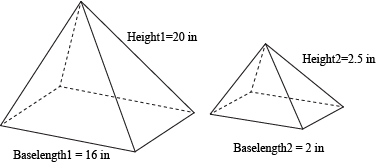# Similar Solid Comparison Worksheets

How to Compare Similar Solids? The solid is anything having volume and surface area. You may have the idea of similar solids. By now, we can compare all such objects if we are able to find their volume and surface area. Here is a way to discover how shapes are similar to each other. Instead of going into the history of complex formulae and fall into confusion, let’s discuss an easier way to compare similar solids. The similar solids may have the same shape and are congruent, but they may differ in size. We go on comparing on their edges instead of how they look. What we need now is a way to relate everything together. We can compare and contrast volumes and surface areas, but we will only get caught in a web of formulas and confusion. Here is an example of the pyramids. If the ratio of measures of the pyramids is the same for all the different measures in both solids, the two are similar.The ratio of the heights should equal the ratio of the base lengths. Basically, every measurement should have the same ratio, called the scale factor.

• ### Basic Lesson

Guides students solving equations that involve an Similar Solid Comparisons. Demonstrates answer checking. If the sides of a cube are multiplied by 4, find the volume of the cube? Let the sides of the stating cube equal 2. The sides of enlarged cube will be 8 (2 times 4).

• ### Intermediate Lesson

Demonstrates how to solve more difficult problems.

• ### Independent Practice 1

A really great activity for allowing students to understand the concept of Similar Solid Comparisons. In a cone, if the radius and the height are 2 times more, what happens to the volume?

• ### Independent Practice 2

Students find the Similar Solid Comparisons in assorted problems. The answers can be found below.

• ### Homework Worksheet

Students are provided with problems to achieve the concepts of Similar Solid Comparisons.

• ### Skill Quiz

This tests the students ability to evaluate Similar Solid Comparisons. In a cone, if the radius and the height are 2 times more, what will happen to the volume?